Following on the initial “PCA Tutorial”, a multivariate dataset will be now used to explore how to conduct Principal Component Analysis [PCA] in R, interpret numerical outputs, and visualize the data. This dataset will also serve to examine some common pitfalls of PCA analyses and common misconceptions regarding PCA interpretations.

### The “Hands” Morphometric Dataset

The dataset used here includes eight linear dimensions recorded in centimeters (morphometric measurments of fingers + height) for nine students and one instructor. The students are anonymous and simply labeled as students 1-9. And given that I was the instructor, the instructor is labeled as ‘me’. In addition, a factor variable ‘gender’ is included, with two levels: ‘female’ and ‘male’. From a technical point of view, this dataset is suitable for PCA because all variables are numerical and continuous, no missing values are present, and there are more observations than variables. In addition, variables are linear biometric measurements and thus expected to correlate tightly but not perfectly. Finally, all numerical values are in the same units (cm).

### Initial assessment and data pre-processing

It is convenient to store numerical variables as a separate object. Here, the object is named ‘handsdata’ and contains only numerical variables that will be used to derive principal components. The one factor available for grouping observations (‘gender’) will be stored as a separate object to be used later for plotting.

hands <- read.csv("sillymorphdata.csv", row.names=1, header=T)  # read raw data
(handsdata <- hands[,1:8])    # subset of data with numeric variables only
##          thumb index middle ring pinky thumbW indexE height
## student1   6.6   7.3    8.4  7.8   6.4    2.3    2.1    184
## student2   5.4   7.1    8.5  7.6   6.5    1.9    1.9    175
## student3   6.3   7.3    7.9  7.4   6.1    2.0    1.8    173
## student4   6.8   7.4    8.3  7.7   6.1    2.3    2.1    170
## student5   6.1   7.4    8.1  7.5   6.1    2.1    2.3    169
## student6   6.0   7.8    8.8  7.9   6.3    2.4    2.0    176
## student7   7.6   8.2    8.6  8.3   6.9    2.7    2.5    185
## student8   6.4   7.2    8.8  7.9   6.4    2.2    2.1    183
## student9   5.6   6.7    7.4  6.5   5.4    1.8    1.6    164
## me         6.6   7.9    8.7  8.3   6.9    2.4    2.3    174
(gender <- as.factor(hands[,9]))        # grouping variable/factor (gender)
##   male   female female male   male   male   male   male   female male
## Levels: female male

Prior to PCA anaysis, a decision must be made to either fully standardize the data (i.e., correlation-based PCA) or only center the data without scaling (i.e., covariance-based PCA). Because all measurements included here are in the same units, variance may be potentially comparable across variables and thus covariance-PCA may be more appropriate. However, in this specific data example variables vary in dispersion with the variable ‘height’ having a dramatically greater variance than any of the finger variables.

round(apply(handsdata, 2, var), 3)
##  thumb  index middle   ring  pinky thumbW indexE height
##  0.394  0.187  0.198  0.265  0.190  0.072  0.069 50.473

Note that the difference between variance of ‘height’ and variance of other variables ranges from 2 to 3 orders of magnitude depending on a specific finger variable. This is really not that surprising, given the humans vary in height by 10s of centimeters but their fingers dimensions vary only by centimeters or less. However, this also means that if we conduct covariance-based PCA on raw centered data, the height variable will overwhelm the analysis. This is indeed the case.

centdata <- scale(handsdata, scale=F)
prcomp(centdata)$rotation[,1:4] # output limited to the first four PCs ## PC1 PC2 PC3 PC4 ## thumb -0.0508 0.552 -0.69591 -0.10501 ## index -0.0306 0.483 0.08899 0.03745 ## middle -0.0451 0.204 0.53426 -0.60731 ## ring -0.0531 0.430 0.33194 -0.01028 ## pinky -0.0449 0.303 0.32941 0.70808 ## thumbW -0.0247 0.259 -0.05573 -0.31326 ## indexE -0.0203 0.258 0.00634 0.13820 ## height -0.9943 -0.101 -0.02276 0.00525 Note that the eigenvector for PC1 has a loading approaching 1 for the ‘height’ variable, whereas absolute values of loadings for all other variables are <0.06*. This means that PC1 is effectively synonymous with ‘height’ and all other variables carry virtually no weight when it comes fo PC1 scores. This is clearly not desirable. As it turns out, for the traditional multivariate morphometrics approach, a standard protocol is to LOGE-transform the variables and then conduct covariance-based PCA. This transformation retains information about variance (and allometry) while minimizing the unwanted effects of variables with high variances. *The eigenvector coefficients (or ‘loadings’) for PC1 produced by prcomp function for this dataset happened to be all negative, but keep in mind that polarity of PC loadings and scores is arbitrary (see the previous PCA tutorial). centlogdata <- scale(log(handsdata), scale=F) # scaling is automatically done by prcomp function used below, so the scale step above is not needed except if we want to see raw data after centering or use them to derive pc scores (see below). pca.res <- prcomp(centlogdata) pca.res$rotation[,1:4]  # output limited to the first four PCs
##           PC1     PC2     PC3     PC4
## thumb  -0.376  0.6828 -0.0532  0.4918
## index  -0.240 -0.0139 -0.1133 -0.3962
## middle -0.188 -0.4141 -0.2736 -0.0208
## ring   -0.294 -0.3014 -0.1816  0.1170
## pinky  -0.272 -0.4218 -0.1404  0.3638
## thumbW -0.531  0.2401 -0.4070 -0.4848
## indexE -0.554 -0.1547  0.7915 -0.0387
## height -0.128 -0.1087 -0.2543  0.4671

When log-transformed data were used to derive a covariance-based PCA ordination, all loadings for PC1 were substantial (i.e., all variables influenced the scores of PC1). All loadings have the same sign indicating positive correlations between all variables. This is actually expected for linear biometric measurements as they all should scale with the overall body size and thus correlate with one another quite tightly. This is indeed the case here, as documented by positive (and generally high) values of Pearson’s correlation coefficients for all variable pairs:

round(cor(centlogdata), 2)
##        thumb index middle ring pinky thumbW indexE height
## thumb   1.00  0.72   0.37 0.65  0.55   0.85   0.73   0.56
## index   0.72  1.00   0.67 0.85  0.79   0.91   0.81   0.51
## middle  0.37  0.67   1.00 0.91  0.85   0.71   0.67   0.73
## ring    0.65  0.85   0.91 1.00  0.95   0.86   0.85   0.74
## pinky   0.55  0.79   0.85 0.95  1.00   0.73   0.78   0.75
## thumbW  0.85  0.91   0.71 0.86  0.73   1.00   0.83   0.65
## indexE  0.73  0.81   0.67 0.85  0.78   0.83   1.00   0.56
## height  0.56  0.51   0.73 0.74  0.75   0.65   0.56   1.00

The eigenvalue of PC1 is much larger than subsequent eigenvalues and consequently most of variance (>80%) is explained by PC1, whereas subsequent PCs account for much less variance (PC2 = 8.6%, PC3 = 5.1%, etc.). This again is not uncommon for traditional morphometric data, where most of the variance is linked to variation in body size and associated allometric shape changes.

round(summary(pca.res)[][2,], 3)
##   PC1   PC2   PC3   PC4   PC5   PC6   PC7   PC8
## 0.817 0.086 0.051 0.025 0.014 0.005 0.001 0.000
# If the above, somewhat more complex, R statement is confusing, explore as follows:
# 1. Run: "str(summary(pca.res))" to examine the structure of this object
# 2. Note that "[]" grabs the 6th list, a matrix-like object
# 3. Try "summary(pca.res)[]"" to see this list
# 4. Note that %variance is stored in the 2nd row of the 6th list. So, [][2,] grabs second row of the 6th list 

### Interpreting Ordinations

The PCA plots typically are ordinations of observations only, rather than biplots, where variables and observation are both projected simultaneously. In the example used here, points on the plots are persons (observations) described by eight morphometric variables (Fig 1). As with all ordinations, the primary interpretation is visual. Points (persons) that plot close to each other are similar in terms of their morphometric variables and those points (persons) that plot far apart differ much more notably in their measurement values. In addition, the ordination shows a clear separation by gender that occurs along the first axis (PC1). Whereas this separation is driven by combined effects of all variables, not all variables are equally important. Highest loadings (eigenvector values for PC1) are associated with index thumb width (‘thumbW’), and index finger width (‘indexE’). Those two variables have loadings with absolute value > 0.5 and their influence on PC1 scores is therefore strongest of all variables. Because loadings are negative for all variables, higher scores on PC1 correspond to lower values for all variables.

par(mfrow=c(1,3), mar=c(5,5,0.1,0.1), pty='square', omi=c(0.5,0.5,0.05,0.05))
mycols <- c('red1', 'blue4')
mycex <- 1.4
PCeig <- 100*round(summary(pca.res)[][2,1:3],3)
for (i in 1:3) {
if (i==1) {a=1; b=2}
if (i==2) {a=1; b=3}
if (i==3) {a=2; b=3}
plot(pca.res$x[,a], pca.res$x[,b], pch=21, las=1, cex=mycex, col=mycols[gender],
xlab=paste('PC', a, ' [', PCeig[a], '%]', sep=''),
ylab=paste('PC', b, ' [', PCeig[b], '%]', sep=''))
}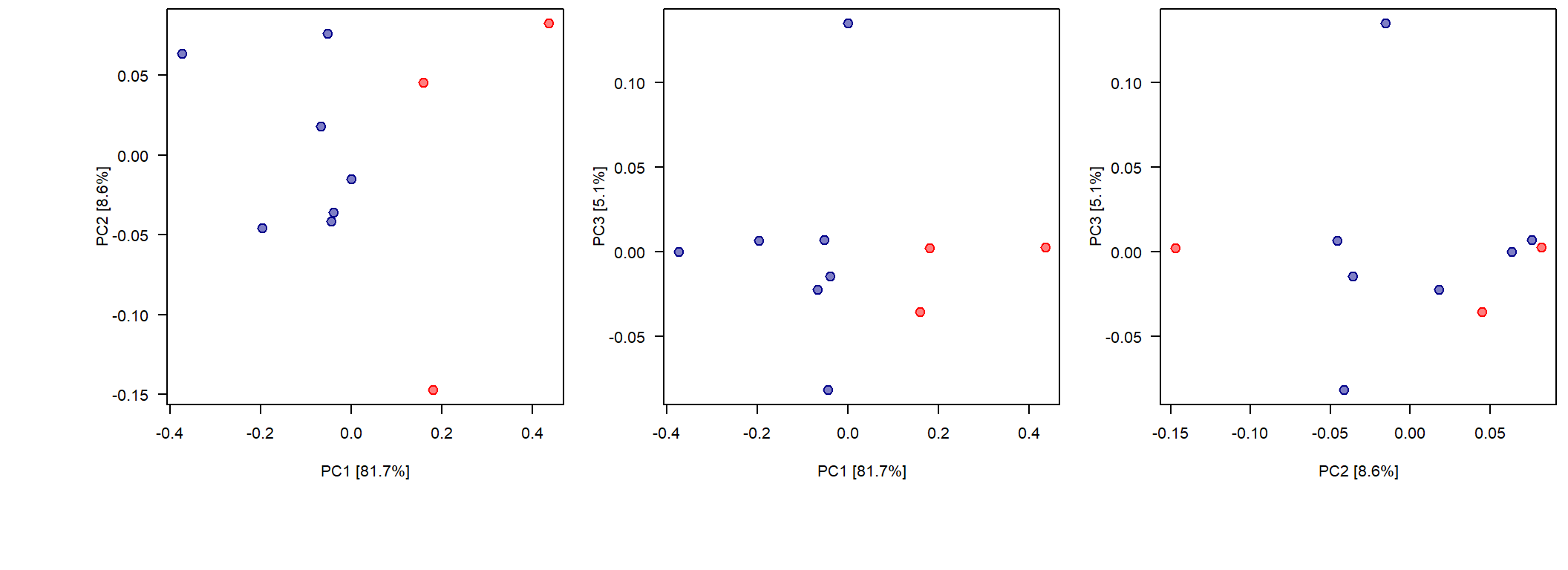Figure 1. Principal component ordination of multivariate morphometric data based on eight linear measurements. Plots show ordinations for the first three PCs, which jointly account for more than 95% of variance in the data.

### Is PCA really a “rigid rotation” that produces “undistorted” ordinations?

PCA is often described as a ‘rigid’ or ‘undistorted’ rotation. However, this is only correct in strict technical terms. It is indeed technically correct to state that multivariate distances between observations are retained after observations are rotated using new axes (PCs). This can be easily demonstrated for the example data used here. The Euclidean distance between observations based on original variables (log-transformed and centered) is the same as the Euclidean distance between observations measured in terms of PC scores. Here are pairwise Euclidean distances between students 1, 2, 3, and 4 computed both ways.

dist(centlogdata[1:4,]) # Euclidean distances between observations based on log-transfromed, centered variables
##          student1 student2 student3
## student2   0.3018
## student3   0.2418   0.2010
## student4   0.0997   0.3271   0.2314
dist(pca.res$x[1:4,]) # Euclidean distances between observations based on pc scores ## student1 student2 student3 ## student2 0.3018 ## student3 0.2418 0.2010 ## student4 0.0997 0.3271 0.2314 So… indeed, distances are the same for variables and PCA scores and thus it is correct to state that PC ordination is a rigid rotation. However, this is somewhat misleading for two reasons. First, typically only the first 2 or 3 PCs are plotted, which means that resulting ordination plots (e.g., PC1 versus PC2) represent 2D or 3D projections of observations. Consequently, the observed relations between observations may be misleading (distorted). This is analogous to interpreting distances between stars by looking up at the night sky, in which case we are actually interpreting a specific 2D projection of stars scattered in three dimensions (the relativity and superstrings theories notwithstanding). That is, points plotting close to each other may be actually further apart depending on their location in other dimensions. The PCA visualization in 2 or 3 dimensions is thus a distorted projection. However, because PC1 and PC2 account for most of the variance in the data, it may often be the case that most observations are close to the plane of the ordination and distortions are trivial. Nevertheless, whereas the PCA rotation may be rigid and undistorted, the 2D visualization such as PC1 x PC2 plots are not faithful representations of the actual multivariate distances between the data. The only exceptions are cases when PCA is applied to two variables (as in the case of a simple major axis regression) and PC1 and PC2 account jointly for 100% of variance in the data - i.e., all observations reside on the plane defined by PC1 and PC2 vectors. Second, we typically rescale axes according to the observed ranges of PC score values. This rescaling may happen when plotting data but may also be implemented as part of PCA by scaling scores for all PC axis to a unit length. Notice that on Figure 1 above, PC1 scores range from -0.4 to 0.4, whereas scores for PC2 and PC3 represent a much narrow range of values. In other words, the ordination plots exaggerated the variance of lower PCs and thus the distances between the points are strongly distorted. A more faitful representation of the data, with all axes scaled the same way (Figure 2), demonstrates that most variation occurs in the direction of PC1, and the inter-point distances in the direction of PC2 and PC3 vectors are much shorter. par(mfrow=c(1,3), mar=c(5,5,0.1,0.1), pty='square', omi=c(0.5,0.5,0.05,0.05)) mycols <- c('red1', 'blue4') mycex <- 1.4 PCeig <- 100*round(summary(pca.res)[][2,1:3],3) for (i in 1:3) { if (i==1) {a=1; b=2} if (i==2) {a=1; b=3} if (i==3) {a=2; b=3} plot(pca.res$x[,a], pca.res$x[,b], pch=21, las=1, cex=mycex, col=mycols[gender], bg=adjustcolor(mycols[gender], 0.5), xlim=c(-0.5, 0.5), ylim=c(-0.5, 0.5), xlab=paste('PC', a, ' [', PCeig[a], '%]', sep=''), ylab=paste('PC', b, ' [', PCeig[b], '%]', sep='')) }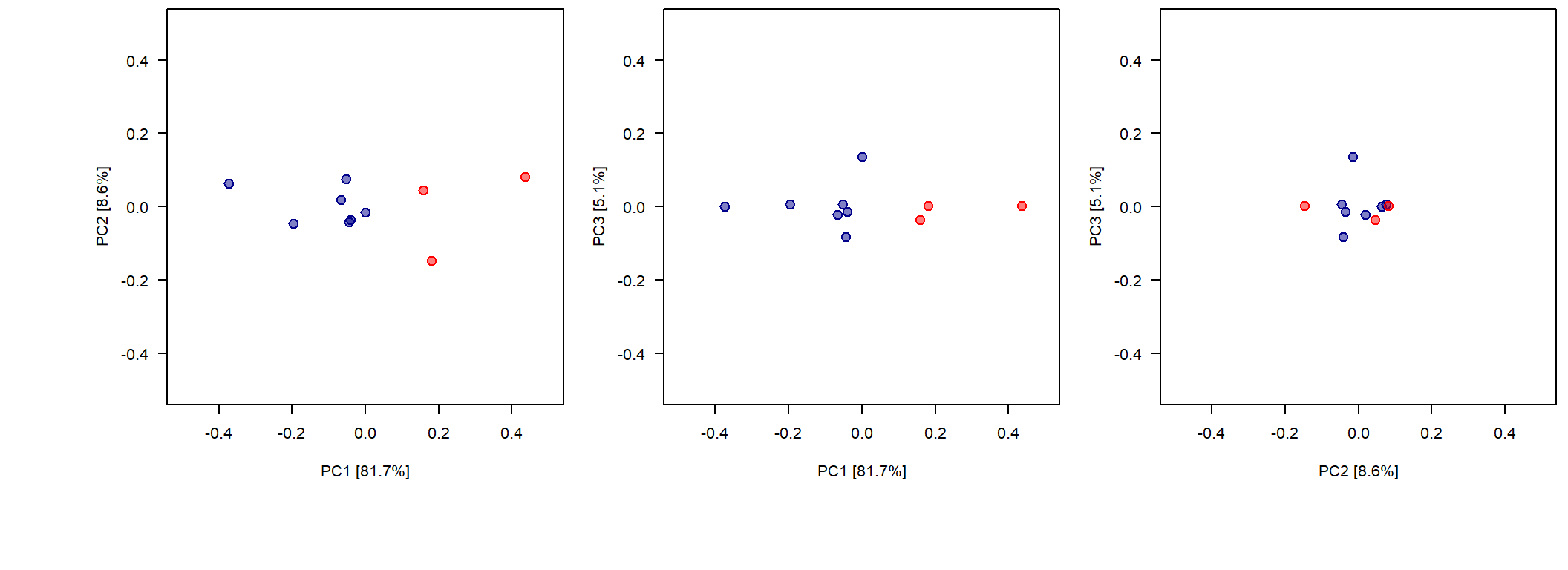Figure 2. Principal component ordination of multivariate morphometric data based on eight linear measurements. Plots show ordinations for the first three PCs, which jointly account for more than 95% of variance in the data. Unlike in the case of Figure 1, this version scaled all axes the same way so the distances between observations are not increasingly exaggerated for higher PCs (PC2 and PC3 axes in this case). In most cases, authors publish PCA ordinations with axes exaggerated. This partly reflects the fact that subtle differences that may exist along PC2 or PC3 can be then visualized more effectively. However, both the authors and the readers should be mindful that the resulting ordinations are distorted despite the fact that PCA is a ‘rigid rotation’ that ‘does not distort distances’. ### Outliers PCA is extremely sensitive to outliers and even a single outlier will dramtically alter the results of the analysis. As an example, let’s make “me” a ‘giant with tiny hands’, so the resulting, modified hands datasest contains one absurd outlier: handsdata2 <- handsdata handsdata2[10,] <- c(0.6, 0.9, 0.7, 0.3, 0.9, 0.04, 0.03, 974.0) pca2 <- prcomp(log(handsdata2)) pca2$rotation[,1:4]
##           PC1       PC2     PC3     PC4
## thumb  -0.288  0.515455 -0.4939  0.0615
## index  -0.257 -0.025960 -0.0588  0.1479
## middle -0.302 -0.306964  0.3215  0.2659
## ring   -0.395 -0.229358  0.1982  0.1857
## pinky  -0.237  0.000373  0.4568  0.0910
## thumbW -0.489  0.263297 -0.1609  0.4324
## indexE -0.516  0.116486  0.1473 -0.8137
## height  0.208  0.709863  0.5948  0.1088

First, note that eigenvector coefficients for PC1 are now dramatically different, with the variable ‘height’ having a postive coefficient and all hand dimensions having negative loadings. Thus, one outlier changed completely the variance-covariance structure of the data.

par(mfrow=c(1,3), mar=c(5,5,0.1,0.1), pty='square', omi=c(0.5,0.5,0.05,0.05))
mycols <- c('red1', 'blue4')
mycex <- 1.4
PCeig <- 100*round(summary(pca2)[][2,1:3],3)
for (i in 1:3) {
if (i==1) {a=1; b=2}
if (i==2) {a=1; b=3}
if (i==3) {a=2; b=3}
plot(pca2$x[,a], pca2$x[,b], pch=21, las=1, cex=mycex, col=mycols[gender],
bg=adjustcolor(mycols[gender], 0.5), xlim=c(-1.5, 8), ylim=c(-1.5, 8),
xlab=paste('PC', a, ' [', PCeig[a], '%]', sep=''),
ylab=paste('PC', b, ' [', PCeig[b], '%]', sep=''))
}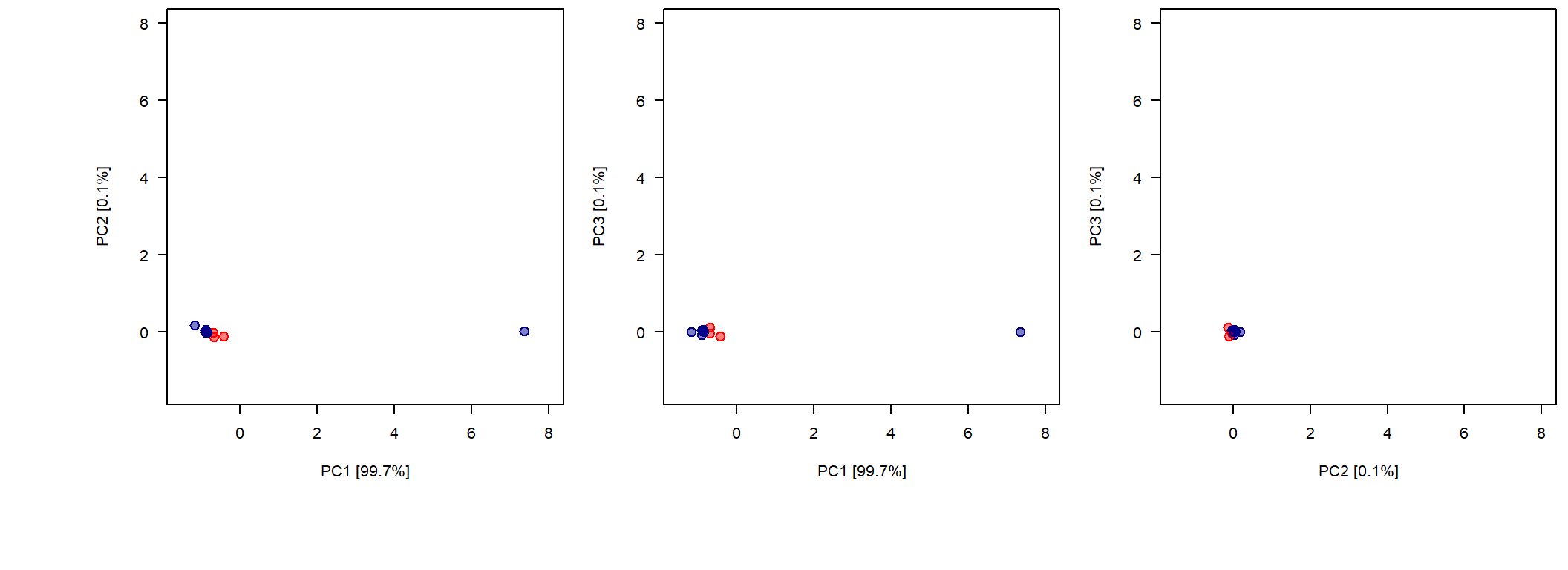Figure 3. A single outlier can dominated the ordination and obscure relations in between all other observations.

Due to that one outlier, a resulting PCA ordination shows that nearly all variation reflects the difference between the outlier and all other observations (Figure 3). This is not incorrect: the outlier is very different after all. However, if we are interested in exploring relations between all observations, that single outlier makes this task nearly impossible. The outlier, by being very far away from all other datapoints, compressed them all into a very small region of the multivariate space. One solution is to remove outliers. The other is to use data transformation/standardization methods that reduce the effect of the extreme values (note that log-transforming was not enough in this specific example). Or, one can consider other ordination techinques that may be less sensitive to outliers.

### A priori and A posteriori scoring

It is critical to stress that PC scores are not comparable across multiple independently derived ordinations. For example, if the morphometric hand data used in the example above were split into two subsets (‘males’ and ‘females’) and analyzed separately, a joint plot of the resulting PC scores is meaningless. Both sets of observations are centered on (0,0) and thus the resulting plot (Figure 4) wrongly indicates that males and females occupy the same region of the morphospace.

outF <- prcomp(log(handsdata[gender=='female',]))
outM <- prcomp(log(handsdata[gender=='male',]))
plot(outF$x[,1], outF$x[,2], pch=16, col='red4', xlab='PC1', ylab='PC2',
ylim=c(-0.15, 0.15), xlim=c(-0.3, 0.3))
points(outM$x[,1], outM$x[,2], pch=16, col='blue3')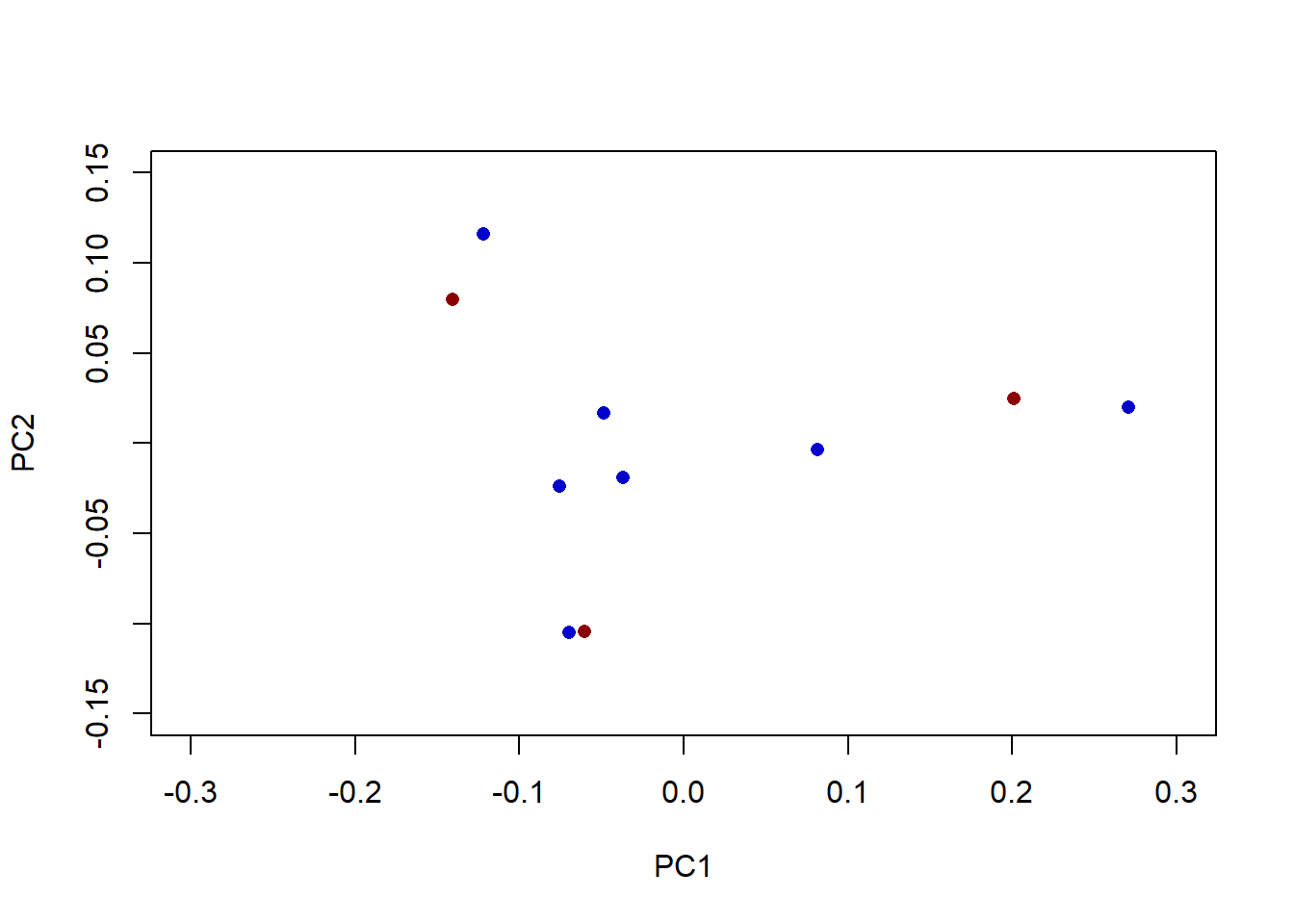Figure 4. A joint plot of PC scores derived separately for males and females wrongly suggests that males and females occupy the same region of morphospace. PC scores derived in separate PCA analyses should not be plotted together or compared directly.

Similarly trivial but important is to distinguish a priori observations that were included in the PCA analysis and thus influenced its outcome from a posterior observations that were scored after PCA analysis was conducted. A posteriori scores can be derived for any observation described by the same set of variables as the observations used originally to derive the ordination. This can be done by matrix multiplication of eigenvectors and observations.

Let’s first show that scores can be re-derived for a priori observations.

outCTL <- prcomp(centlogdata) # PCA for logged and centered data
outCTL$x[1:3,1:3] # scores for the first 3 PCs for the first three observations ## PC1 PC2 PC3 ## student1 -0.0668 0.0181 -0.02221 ## student2 0.1798 -0.1471 0.00205 ## student3 0.1587 0.0452 -0.03538 (centlogdata %*% outCTL$rotation)[1:3,1:3] # same can be derived as matrix product 
##              PC1     PC2      PC3
## student1 -0.0668  0.0181 -0.02221
## student2  0.1798 -0.1471  0.00205
## student3  0.1587  0.0452 -0.03538

For a posteriori plotting it is a bit more complicated because we need to derive centered values for a posteriori observations. For example, let’s exclude “females” from the original PCS analysis and then project those “female” observations a posteriori.

centmale <- scale.default(log(handsdata[gender=='male',]), scale=F) # first the male data have to be scaled
cent.coeff <- attributes(centmale)[] # centering coeff. should be stored for rescaling 'female' values later
pcaMALE <- prcomp(centmale)             # PCA for males
pcaMALE$x[,1:2] # PCA scores for males ## PC1 PC2 ## student1 -0.0371 -0.01929 ## student4 -0.0486 0.01651 ## student5 -0.1219 0.11593 ## student6 -0.0696 -0.10528 ## student7 0.2709 0.01981 ## student8 -0.0753 -0.02412 ## me 0.0816 -0.00357 males.recenter <- as.matrix(log(handsdata[gender=='male',]) - matrix(cent.coeff, nrow(centmale), length(cent.coeff), byrow=T)) (males.recenter %*% pcaMALE$rotation)[,1:2] # PCA scores for males reconstructed correctly
##              PC1      PC2
## student1 -0.0371 -0.01929
## student4 -0.0486  0.01651
## student5 -0.1219  0.11593
## student6 -0.0696 -0.10528
## student7  0.2709  0.01981
## student8 -0.0753 -0.02412
## me        0.0816 -0.00357
females <- log(handsdata[gender=='female',])
females.recenter <- as.matrix(females) - matrix(cent.coeff, nrow(females), length(cent.coeff), byrow=T)
PCFem <- (females.recenter %*% pcaMALE$rotation)[,1:2] # PCA scores for females PCFem ## PC1 PC2 ## student2 -0.299 -0.03630 ## student3 -0.243 -0.02495 ## student9 -0.517 0.00203 Let’s now compare visually the ordinations of the same 10 observations conducted in various ways (Figure 5). par(mfrow=c(1,3), mar=c(5,5,1,0.1), pty='square', omi=c(0.5,0.5,0.05,0.05)) mycols <- c('red1', 'blue4') mycex <- 1.4 plot(pca.res$x[,1], pca.res$x[,2], pch=21, las=1, cex=mycex, col=mycols[gender], bg=adjustcolor(mycols[gender], 0.5), xlab='PC1', ylab='PC2') mtext(side=3, line=-1.2, adj=0.05, cex=0.9, 'A') mtext(side=3, line=0.5, cex=0.7, 'Standard A Priori Analysis of All Observations') plot(outF$x[,1], outF$x[,2], pch=21, col=mycols, xlab='PC1', ylab='PC2', bg=adjustcolor(mycols, 0.5), ylim=c(-0.15, 0.15), xlim=c(-0.2, 0.3)) points(outM$x[,1], outM$x[,2], pch=21, col=mycols, bg=adjustcolor(mycols, 0.5)) mtext(side=3, line=-1.2, adj=0.05, cex=0.9, 'B') mtext(side=3, line=0.5, cex=0.7, 'Incorrect Analysis: A joint plot from two separate PCAs') plot(pcaMALE$x[,1], pcaMALE$x[,2], pch=21, col=mycols, xlab='PC1', ylab='PC2', bg=adjustcolor(mycols, 0.5), ylim=c(-0.15, 0.15), xlim=c(-0.6, 0.3)) points(PCFem[,1], PCFem[,2], pch=21, col=mycols, bg=adjustcolor(mycols, 0.5)) mtext(side=3, line=-1.2, adj=0.05, cex=0.9, 'C') mtext(side=3, line=0.5, cex=0.7, 'A Poteriori Projection of Females on the PC plot for Males')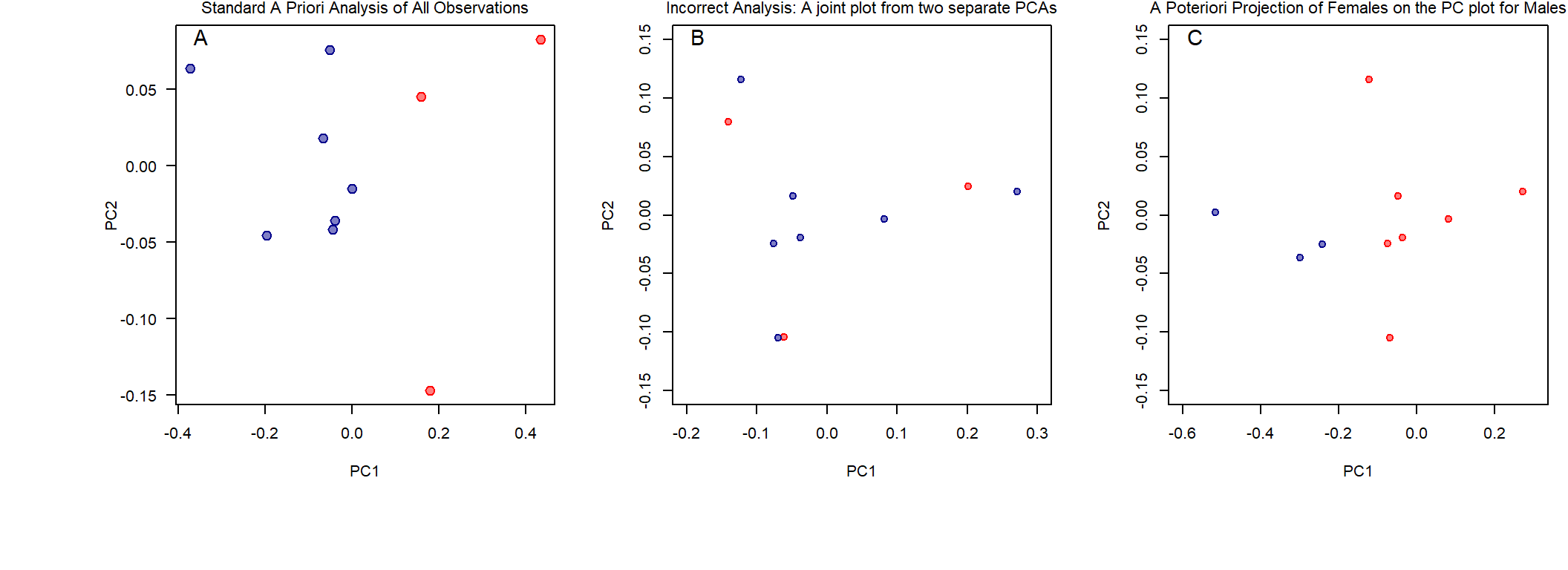Figure 5.Three plots of the PC1 x PC2 ordination for the same 10 observations using different data processing strategies. A. Standard PCA analysis with all 10 observations included a priori. B. Incorrect PCA analysis in which female and male observations were analyzed separately (two independent PCAs) and then plotted jointly. This is meaningless because PC scores are centered and scaled for each analysis, and thus, the scores cannot be meaningfully compared across multiple PC analyses. C. A posteriori analysis, where PCA was derived for males only and then female scores were computed afterwards. Note that this is not synonymous with standard analysis shown on inset ‘A’. Also, note that relation between ‘male’ observations is not the same for plots ‘A’ and ‘C’ (i.e., exclusion of females affected the ordination of males). Whereas typically all observations are included a priori, there exist situations when a posteriori projections of observations can be useful. In the example above (Figure 5), the common approach would be to include all observations a priori (Figure 5A), but as illustrated below there are situations when a posteriori projections can be useful. ### Correlation/Loading Vector Plots and Biplots There are various additional ways to enhance interpretations of PC ordinations. First, we can create a vector plots, representing correlations between PCs and original variables. par(pty='square') plot(0,0, xlim=c(-1.2,1.2), ylim=c(-1.2,1.2), las=1, xlab='PC1', ylab='PC2') for (i in 1:8) { r1 <- cor(centlogdata[,i], pca.res$x[,1])
r2 <- cor(centlogdata[,i], pca.res$x[,2]) arrows(0, 0, r1, r2, length=0.1, col='green4') text(r1, r2, colnames(centlogdata)[i], pos=2, cex=0.6, col='green4') }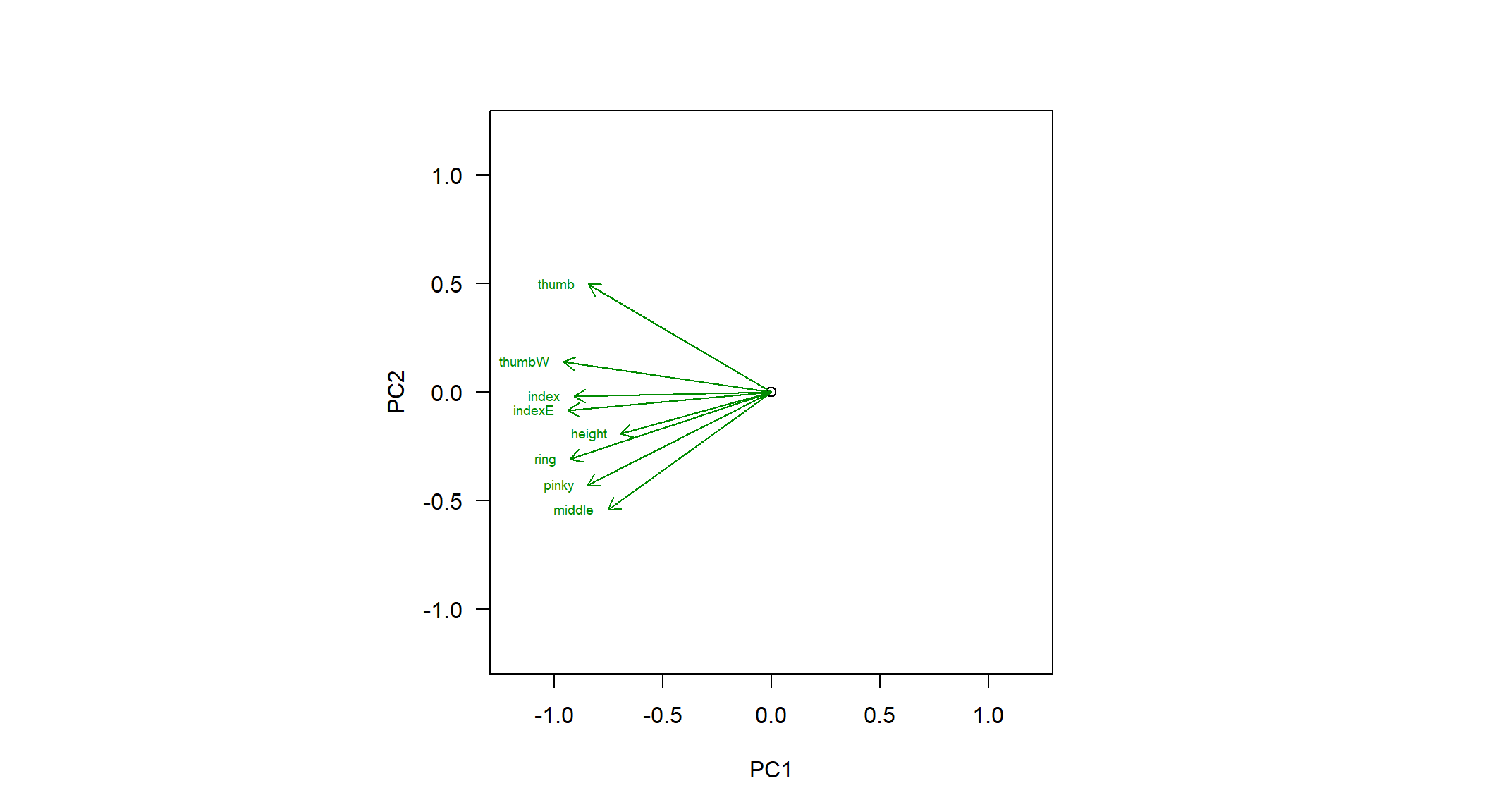Figure 6. Vector plot of Pearson’s correlation coefficients of the eight original variables versus the first two principal components. All vectors show strong negative correlation with PC1 suggesting that PC1 may represent an allometric size vector (Figure 6). Thus, negative scores generally will indicate persons with longer and especially thicker fingers. PC2 on the other hand is correlated with only some variables. In some cases, the correlation is positive (as in the case of the thumb length), in some cases negative (e.g., pinky and middle fingers), and in some cases correlation is approaching zero (e.g., index finger and thumb width). Instead of plotting Pearson’s correlation coefficients, many authors plot vector loadings (Figure 7). par(pty='square') plot(0,0, xlim=c(-0.7,0.7), ylim=c(-0.7,0.7), las=1, xlab='PC1', ylab='PC2') for (i in 1:8) { r3 <- pca.res$rotation[i,1]
r4 <- pca.res$rotation[i,2] arrows(0, 0, r3, r4, length=0.1, col='red3') text(r3, r4, colnames(centlogdata)[i], pos=2, cex=0.6, col='red3') }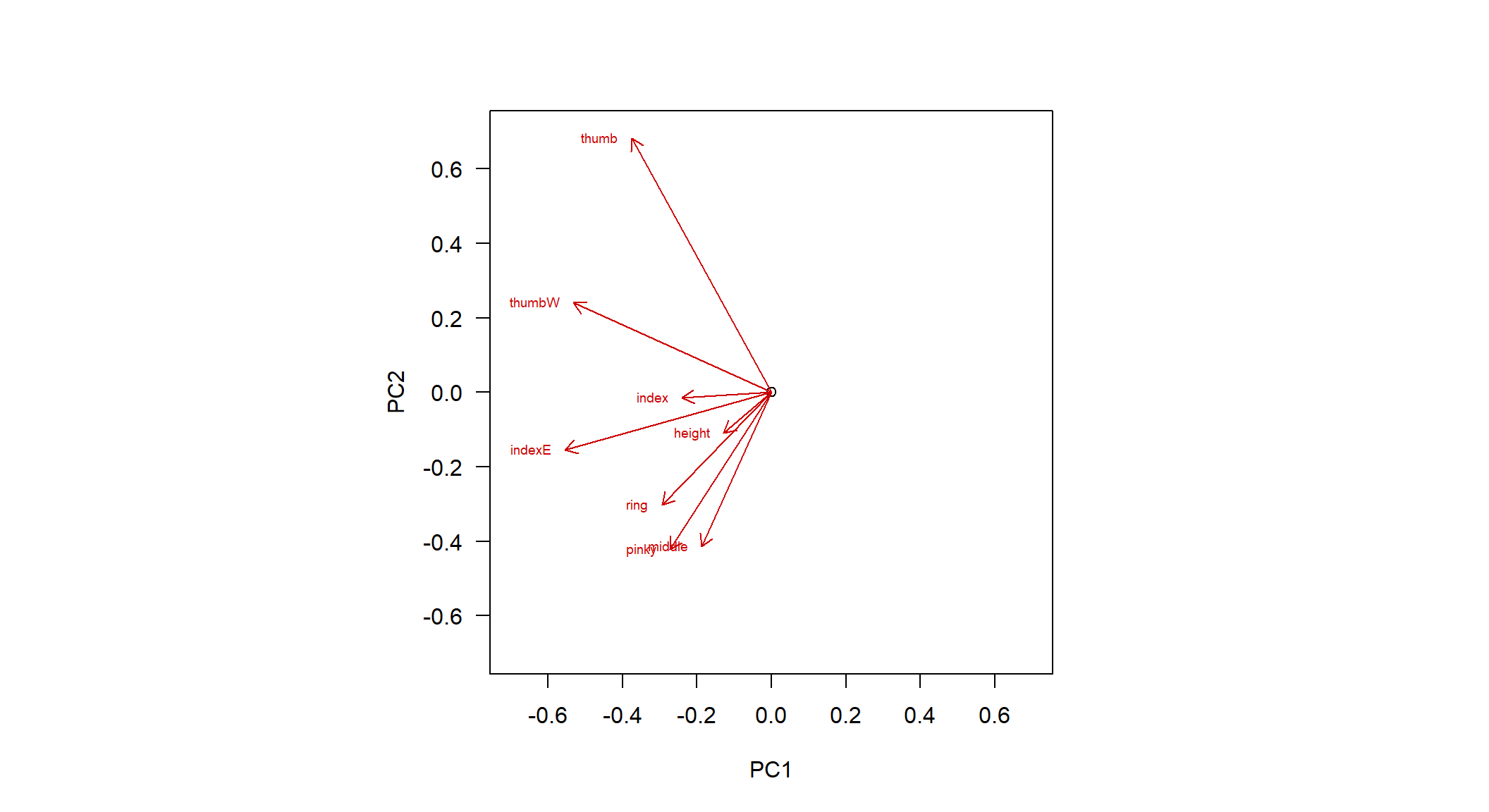Figure 7. Vector plot of loadings of the eight original variables for the first two principal components. Vector plots of loadings (Figure 7) convey similar information to the correlation plot (Figure 5), but the magnitude of values represents the weight/influence of a variable on PC scores rather than strength of correlation between PCs and variables. Finally, variables can be represented as points on the plot, where PC scores of variables are calculated by weighted averaging (as in the package ‘vegan’). This plot (Figure 8) is effectively a biplot similar to those often used to visualize results of Correspondence Analysis or Nonmetric Multdimensional Scaling. par(pty='square') # NOT RUN: install.packages('vegan') # install package 'vegan' if needed library(vegan) vegpca <- rda(centlogdata ~ 1) plot(vegpca$CA$u[,1:2], pch=21, las=1, col=mycols[gender], bg=adjustcolor(mycols[gender], 0.5), xlim=c(-0.8, 0.8), ylim=c(-0.8, 0.8)) points(vegpca$CA$v[,1:2], pch='+') text(vegpca$CA$v[,1:2], rownames(vegpca$CA$v), pos=1, cex=0.8)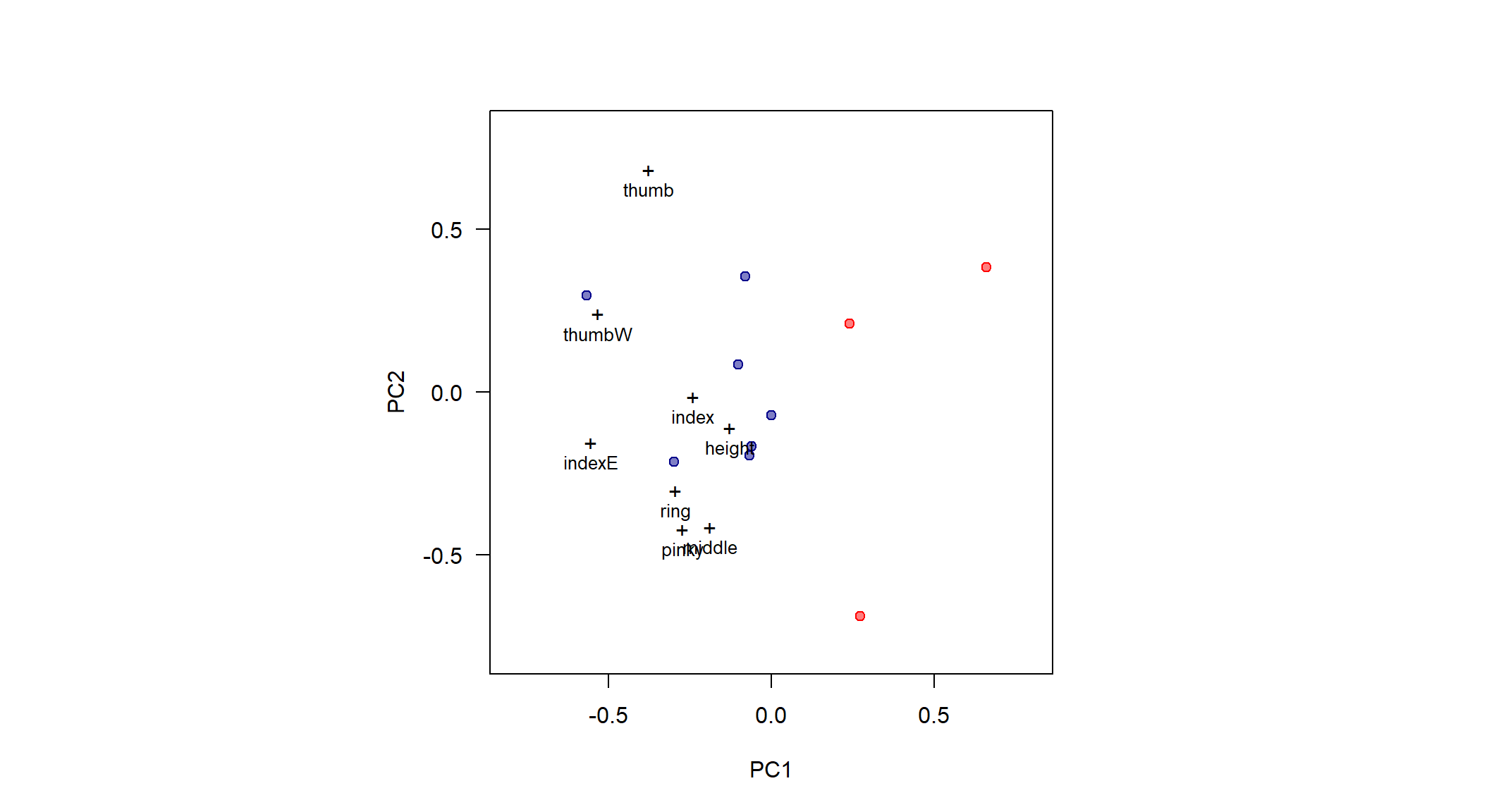Figure 8. Biplot of 10 observations (color-coded as red=females and blue=males) and eight original variables (+ signs). The PC scores of variables were derived by weighted averaging of variable scores (vegan package, rda function). Note that scores of variables are easily derived using matrix algebra functions available in R. a1 <- vegpca$CA$v[,1:2] # variable scores for PC1 and PC2 in vegan a1 # variable scores produced by vegan represent rescaled scores calculated via weighted averaging  ## PC1 PC2 ## thumb -0.376 0.6828 ## index -0.240 -0.0139 ## middle -0.188 -0.4141 ## ring -0.294 -0.3014 ## pinky -0.272 -0.4218 ## thumbW -0.531 0.2401 ## indexE -0.554 -0.1547 ## height -0.128 -0.1087 a2 <- t(centlogdata)%*%pca.res$x[,1:2]
a3 <- c(a1[,1]/a2[,1], a1[,2]/a2[,2]) # find scaling coefficient used by rda
a2*a3 # one can see that species scores are simply weighted averaged scores multiplied by a constant
##           PC1     PC2
## thumb  -0.376  0.6828
## index  -0.240 -0.0139
## middle -0.188 -0.4141
## ring   -0.294 -0.3014
## pinky  -0.272 -0.4218
## thumbW -0.531  0.2401
## indexE -0.554 -0.1547
## height -0.128 -0.1087

### A posteriori projections of synthetic data

For some types of data, we can further explore the PC ordination by creating synthetic observations that vary in some predictable manner. Plotting such synthetic data can help us understand the ordination space in a more intuitive manner. The morphometric hand data used here offer a suitable example. To explore PC1 x PC2 ordination space, we can create artificial hands that vary in size but not in shape. For example, we can take ‘student 1’ and create a whole series of artificial, isometrically-scaled humans by multiplying all variables by the same constant (e.g., 0.9 to make the student smaller or 1.02 to make the student a tiny bit larger).

real.data <- scale(log(handsdata), scale=F)
pcaREAL <- prcomp(real.data)
scale.cf <- attributes(real.data)[]

# artificial data
shape1 <- handsdata[1,]
shape2 <- handsdata[10,]
isorange <- seq(0.9, 1.02, 0.01)
cex1a <- isorange * as.numeric(shape1)
cex2a <- isorange * as.numeric(shape2)
art.data1 <- NULL;  for (i in isorange) art.data1 <- rbind(art.data1, shape1*i)
art.data2 <- NULL;  for (i in isorange) art.data2 <- rbind(art.data2, shape2*i)
art.out1 <- as.matrix(log(art.data1) - matrix(scale.cf, nrow(art.data1), length(scale.cf), byrow=T))
art.out2 <- as.matrix(log(art.data2) - matrix(scale.cf, nrow(art.data2), length(scale.cf), byrow=T))
art.sc1 <- art.out1%*%pcaREAL$rotation art.sc2 <- art.out2%*%pcaREAL$rotation
plot(pcaREAL$x[,1], pcaREAL$x[,2], pch=21, col=mycols[gender], xlab='PC1', ylab='PC2',
bg=adjustcolor(mycols[gender], 0.5), ylim=c(-0.15, 0.15), xlim=c(-0.3, 0.3))
points(art.sc1[,1], art.sc1[,2], type='o', pch=21, cex=log10(cex1a - 150), col='green')
points(art.sc2[,1], art.sc2[,2], type='o', pch=21, cex=log10(cex2a - 150), col='green3')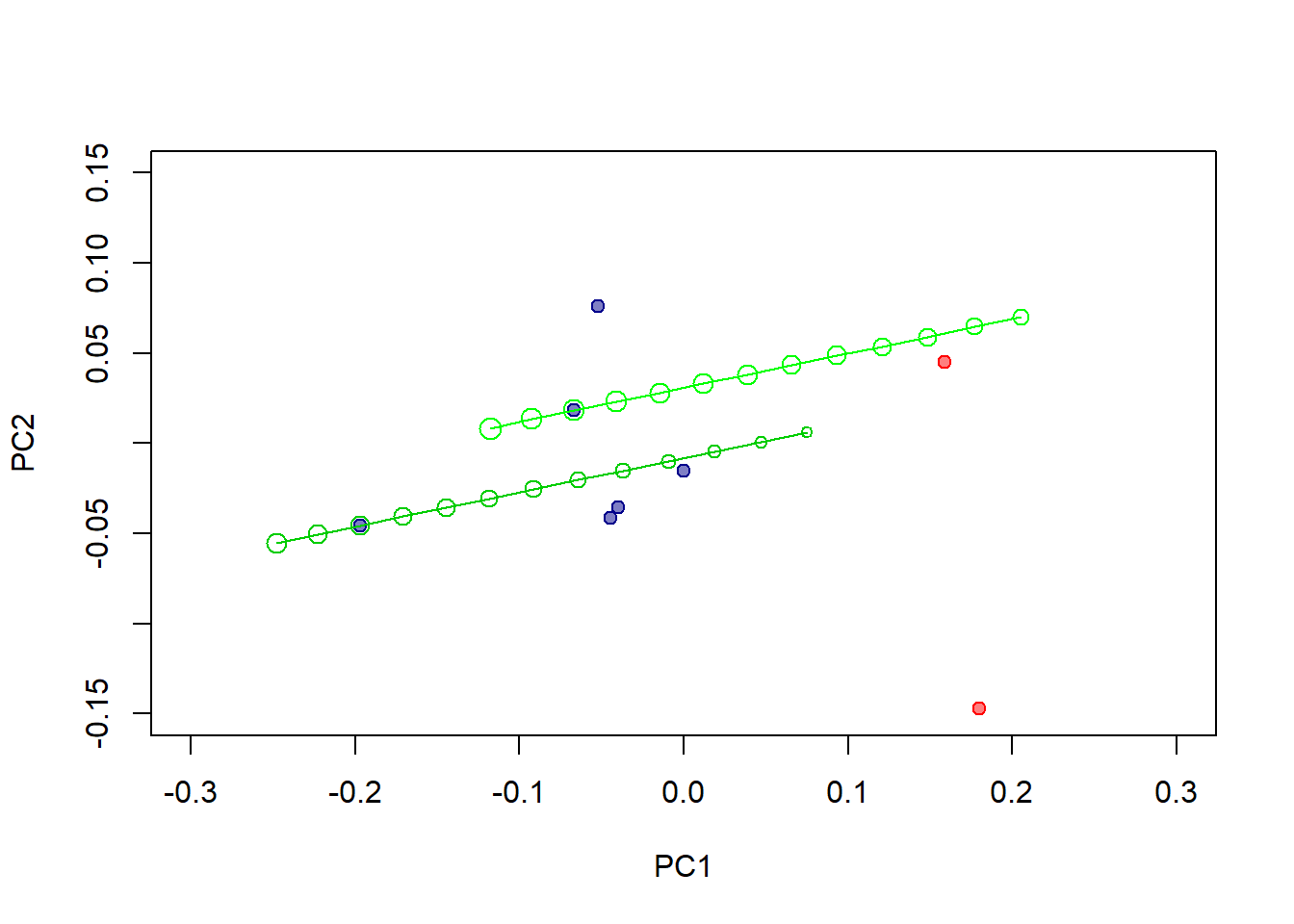Figure 9. A posteriori projection of synthetic observations can be used to aid an intuitive interpretation of PCA ordinations. Here, linear measurements of ‘Student 1’ and ‘me’ (the two arbitrarily selected observations) were scaled sequentially to create a series of artificial humans that are isometric replicas of the two selected persons. Size of symbols reflects body height.

The projection above (Figure 9) helps to understand the PC1 vs. PC2 plot better. Isometric arrays of synthetic persons form lines that are aligned somewhat obliquely with PC1. Clearly, PC1 is not an isometric size axis, but rather reflects increase in size as well as associated shape changes and likley other sources of variation. Note that for the two “shapes” (‘student 1’ and ‘me’), synthetic humans of the same height (visualized by symbol size) do not have the same PC1 scores. Indeed, as suggested by Figures 6-8, the variable ‘height’ correlates relatively weakly with PC1. In other words, PC1 may be a good predictor of ‘latent allometric size’ but not really a useful proxy of height (that is, for this specific dataset, PC1 scores will increase with height for any “isometrically grown” human, but for real allometric humans that differ in shapes, PC1 is a poor predictor of height).

### Final Remarks

Principal Component Analysis - including its interpretations, strengths, and pitfalls - is a major topic that cannot be covered exhaustively in a single tutorial. Hopefully, the simple examples provided above will offer new PCA users a useful starting point to explore this topic further.